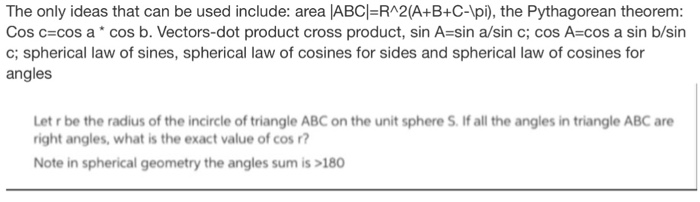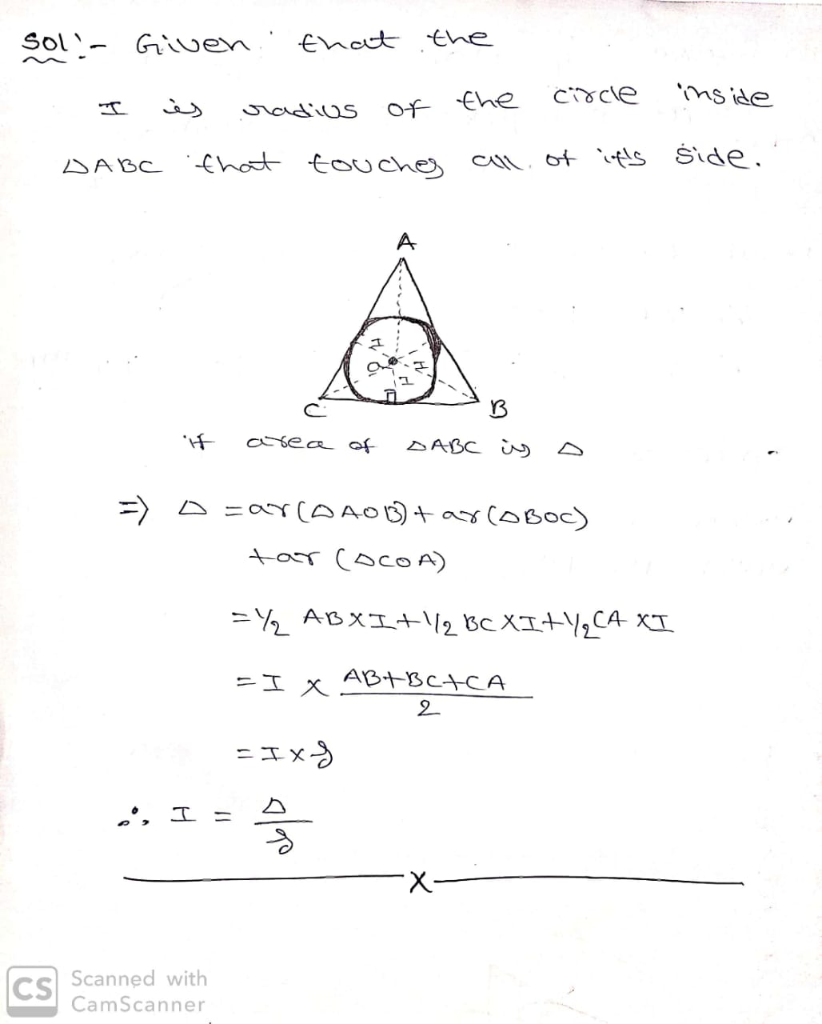# The only ideas that can be used include: area ABCI-RA2(A+B+C-lpi), the Pythagorean theorem: Cos c cos a cos b. Vectors-dot product cross product, sin A-sin a/sin c; cos A-cos a sin b/sin c; spher...The only ideas that can be used include: area ABCI-RA2(A+B+C-lpi), the Pythagorean theorem: Cos c cos a cos b. Vectors-dot product cross product, sin A-sin a/sin c; cos A-cos a sin b/sin c; spherical law of sines, spherical law of cosines for sides and spherical law of cosines for angles Let r be the radius of the incircle of triangle ABC on the unit sphere S. If all the angles in triangle ABC are right angles, what is the exact value of cos? Note in spherical geometry the angles sum is >180##### Add Answer of: The only ideas that can be used include: area ABCI-RA2(A+B+C-lpi), the Pythagorean theorem: Cos c cos a cos b. Vectors-dot product cross product, sin A-sin a/sin c; cos A-cos a sin b/sin c; spher...
More Homework Help Questions Additional questions in this topic.